Navigation Panel:(These buttons explained below)Question Corner and Discussion Area

# Applications of the Geometric Mean

Asked by Senthil Manick on May 22, 1997:
When would one use the geometric mean as opposed to arithmetic mean? What is the use of the geometric mean in general?
The arithmetic mean is relevant any time several quantities add together to produce a total. The arithmetic mean answers the question, "if all the quantities had the same value, what would that value have to be in order to achieve the same total?"

In the same way, the geometric mean is relevant any time several quantities multiply together to produce a product. The geometric mean answers the question, "if all the quantities had the same value, what would that value have to be in order to achieve the same product?"

For example, suppose you have an investment which earns 10% the first year, 50% the second year, and 30% the third year. What is its average rate of return? It is not the arithmetic mean, because what these numbers mean is that on the first year your investment was multiplied (not added to) by 1.10, on the second year it was multiplied by 1.60, and the third year it was multiplied by 1.20. The relevant quantity is the geometric mean of these three numbers.

The question about finding the average rate of return can be rephrased as: "by what constant factor would your investment need to be multiplied by each year in order to achieve the same effect as multiplying by 1.10 one year, 1.60 the next, and 1.20 the third?" The answer is the geometric mean. If you calculate this geometric mean you get approximately 1.283, so the average rate of return is about 28% (not 30% which is what the arithmetic mean of 10%, 60%, and 20% would give you).

Any time you have a number of factors contributing to a product, and you want to find the "average" factor, the answer is the geometric mean. The example of interest rates is probably the application most used in everyday life.

Here are some basic mathematical facts about the arithmetic and geometric mean:

Suppose that we have two quantities, A and B. Taking their arithmetic mean we get the number (A+B)/2 which can be interpreted in a number of ways. One interpretation (probably the most common) is that this quantity is the midpoint of the two numbers viewed as points on a line.

Now suppose that we have a rectangle with sides of lengths A and B. The arithmetic mean can also be interpreted as the length of the sides of a square whose perimeter is the same as our rectangle. Similarly, the geometric mean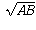is the length of the sides of a square which has the same area as our rectangle.

It is known that the geometric mean is always less than or equal to the arithmetic mean (equality holding only when A=B). The proof of this is quite short and follows from the fact that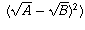is always a non-negative number. This inequality can be surprisingly powerful though and comes up from time to time in the proofs of theorems in calculus.

Asked by G. Ellis, student, Southeast Bulloch High on January 16, 1997:
Could you give the formula for the geometric mean for a series of numbers if I am trying to get the compound annual growth rate for a series of number that include negative numbers?
In general, you can only take the geometric mean of positive numbers. The geometric mean of numbersis the nth root of the product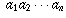.

In your example, you are taking the mean of positive numbers. For example, if you're looking at an investment that increases by 10% one year and decreases by 20% the next, the simple rates of change are 10% and -20%, but that's not what you're taking the geometric mean of.

At the end of the first year you have 1.1 times what you started with (the original plus another tenth of it). At the end of the second year you have 0.8 times what you started the second year with (the original minus one fifth of it). So, the numbers you are taking the geometric mean of are 1.1 and 0.8. This mean is approximately 0.938.

This means that, on average, your investment is being multiplied by 0.938 (= 93.8%) each year, a 6.2% loss.

So, the compound anual growth rate is (approximately) -6.2%.

Asked by Paul van Esbroeck on October 5, 1997:

(Question abridged from original posting)

I am presently engaged in a dispute with a bank concerning a Stock Market Tracker GIC. I have found significantly different definitions for the terms "average percentage growth" and "average percentage growth rate", and in many instances I cannot distinguish between the use of the words "growth" vs. "growth rate" in the literature. It was in investigating growth that I came to your question page about the geometric mean.

While I, like most people I asked, originally understood these GICs to be guaranteeing a rate of return equal to the rate of growth of the index, the bank has a different interpretation.

Let's say a stock market index starts at 1000, and at the end of 1 month is 1010, at the end of 2 months is 1020, and so on, ending up at 1120 after one full year.

The bank seems to be considering the growth by month end for each month (which is 10 for the first month, 20 for the second, and so on, with this growth being 120 by the end of the twelfth month), then averaging these growths, obtaining an "average growth" of 60 and an average percentage growth of 60/1000 or 6%.

For other definitions there seems to be quite a bit of agreement that:

• The real growth was 120/1000 = 12%.
• The average percentage growth rate is 12% per year.
• The annual compound growth rate is 12%.

It seems to me that it is incorrect to average growth as done by the bank. Since an average should be of equal periods, in the banks formula we average growth for the first month with growth for the first 11 months. Since the growth was 10 index units each month, should the average growth not be 10 units per month, but how is this different from the average growth rate?

What if not growth, do you call the plot of (Index value minus Index value at some starting time) ? What if any, is the correct distinction between growth and growth rate ? Do you find the term "average percentage growth" as used by the bank problematic?

First let's try to get the words sorted out. Then we can address the situation you describe.

The word "growth" is often used quite loosely to mean any of the above concepts. The best and most correct definition of growth would be a quantity that is associated to a particular period in time, and describes the index value at the end of the period minus the index value at the beginning of the period. In your example, the growth for the period consisting of the first month was 10, and the growth for the period consisting of the first 12 months was 120.

"Growth rate" describes growth per unit time. It may vary with time. The "average growth rate" over a period is the growth divided by the length of the period. If the growth rate is constant over a period, then the average growth rate over the period will be the same as that constant value.

In your example the growth rate was a constant 10 units per month. The average growth rate over every period was also 10 units per month. For example, the average growth rate during the first month was 10 units per 1 month = 10 units per month. The average growth rate during the entire year was 120 units per 12 months = 10 units per month.

These concepts are not relevant to typical investements, stock market indices, etc., because the growth rate tends to be proportional to the index value. After all, if a 10 dollar investment grew by 1 dollar over a year (an average growth rate of 1 dollar per year), you'd expect a 10,000 dollar investment to grow by 1000 dollars per year: a very different growth rate!

So instead, the relevant concepts are percentage growth and growth rates. The percentage growth over a period is the ratio of the growth to the starting value. In your example, the percentage growth during the first month was 1%. The percentage growth during the second month was about 0.99% (10/1010). The percentage growth during the entire year was 12% (120/1000).

The percentage growth rate is the ratio of the growth rate to the current value. Thus, if an index value is 1000 and it is growing at 10 units per month, it is experiencing a percentage growth rate of 1% per month. If its value is 1010 and it is growing at 10 units per month, its percentage growth rate is about 0.99% per month. And so on. In your exaple, by the end of the year it is still growing at 10 units per month and its value is 1120, so the percentage growth rate by the end of the year has dropped to about 0.89%.

The only tricky thing about percentage growth rate is in changing units: a percentage growth rate of 1% per month is not the same thing as 12% per year. If an index were growing at a constant percentage growth rate of 1% per month, after one month it would be 1.01 times its starting value, after two months it would be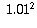times its starting value, and after 12 months it would be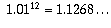times its starting value.

Therefore, a percentage growth rate of 1% per month is the same as a percentage growth rate of about 12.7% per year.

If the percentage growth is G over a period of T time units, then the average percentage growth rate over that period is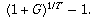To figure out the average percentage growth rate of your example over the entire year: if your units are years, then T=1. G = 12%, so the average percentage growth rate is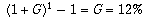per year. If your units are months, the average percentage growth rate is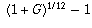which is about 0.949% per month. These are each saying the same thing; an average percentage growth rate of 12% per year is the same as an average percentage growth rate of about 0.949% per month.

Often, in investment circles, when people refer to "growth" or "growth rate" they are meaning percentage growth and percentage growth rate.

Now, on to your particular situation.

As you point out, it is not particularly meaningful to average the growths over time periods of wildly differing lengths. So, to average the growth during the first month with the growth during the first 12 months is not a reasonable thing to do.

However, what is reasonable to do is to average the growths over overlapping time periods of similar lengths, to smooth out fluctuations in the index. For example, let's suppose the end of the year happened to be a really bad day on the stock market, and instead of rising to 1120 the index fell back to 1000, jumping back up to 1120 the next day. Would you really want to say that there was no growth at all during the year, just because the day you picked to evaluate the index happened to be a bad one? I think not.

So, what is commonly done is to average the index value over a certain time period. For example, while what you describe is unreasonable, it could be perfectly reasonable to say that the GIC's rate of return from 1996 to 1997 will be the percentage growth of the index's average 1996 value to its average 1997 value. That way, fluctuations due to the particular day the index is measured will be smoothed out.

Now, if the index were a constant 1000 during all of 1996, and rose to 1120 in the manner you describe during 1997, you would unfortunately only be getting a 6% return from average 1996 value (1000) to average 1997 value (1060). But that's because some of the 1996 behaviour is being factored in as well.

Another thing that could be reasonable is to average the percentage growths over different 12-month periods ending in the same year. For example, you could take the January 1996-January 1997 percentage growth, the February 1996-February 1997 percentage growth, and so on, and average them. This is a reasonable sort of average to take, since the percentage growths are being averaged over equal but overlapping periods.

These sorts of averages have the advantage of smoothing out wild fluctuations in the index. For example, if the index started 1996 at 1000 and rose at 10 units per month steadily, ending 1997 at almost 1240 but plummeting down to 1150 on the last day of 1997 due to a temporary correction in the markets, you would still get the benefit of the growths. Even though the December 31, 1996 to December 31, 1997 growth was from 1120 to 1150, just 2.7% on the entire year, the November 30, 1996 to November 30, 1997 growth was from 1110 to 1230, about 11%. So, by including these in the average, rather than just looking at the average percentage growth over a single year-long period, you get a much less volatile measure of performance.

My suspicion is that the GIC you are concerned about probably employs some sort of legitimate averaging such as the above, and that the bank has miscommunicated the calculation method to you.

Another possibility is that the bank may be measuring index value from start of investment to the average index value during the year preceding the end of investment. That means that during the first year of the investment you have the unreasonable calculation you described, but over the long term those effects are negligble. For instance, the 10-year return might be the average of the index return over the twelve periods all beginning on the date of invesment and ending on the twelve month-end dates during that 10th year. True, this is still averaging growth over periods of differing length, but the lengths range from 9 years to 10 years and this is much less of a difference than your example where they range from 1 month to 12 months!

I will not venture to speculate further on what methods the bank may or may not be using for the GIC you have in mind. However, I hope this clears up for you some of the mathematical ideas involved.

[ Submit Your Own Question ] [ Create a Discussion Topic ]

This part of the site maintained by (No Current Maintainers)
Last updated: April 19, 1999
Original Web Site Creator / Mathematical Content Developer: Philip Spencer
Current Network Coordinator and Contact Person: Joel Chan - mathnet@math.toronto.edu

Navigation Panel: